# Algebra 1 : How to find the solution for a system of equations

## Example Questions

1 2 3 4 5 7 Next →

### Example Question #92 : Equations / Solution Sets

A blue train leaves San Francisco at 8AM going 80 miles per hour. At the same time, a green train leaves Los Angeles, 380 miles away, going 60 miles per hour. Assuming that they are headed towards each other, when will they meet, and about how far away will they be from San Francisco?

Possible Answers:

Around 3AM the next day, about 1,520 miles away from San Francisco

Around 2:45AM, about 200.15 miles away from San Francisco

Around 10:43AM, about 217.12 miles away from San Francisco

The two trains will never meet.

Correct answer:

Around 10:43AM, about 217.12 miles away from San Francisco

Explanation:

This system can be solved a variety of ways, including graphing. To solve algebraically, write an equation for each of the different trains. We will use y to represent the distance from San Francisco, and x to represent the time since 8AM.

The blue train travels 80 miles per hour, so it adds 80 to the distance from San Francisco every hour. Algebraically, this can be written as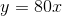.

The green train starts 380 miles away from San Francisco and subtracts distance every hour. This equation should be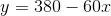.

To figure out where these trains' paths will intersect, we can set both right sides equal to each other, since the left side of each is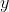.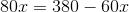add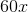to both sides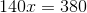divide both sides by 140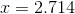Since we wrote the equation meaning time for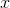, this means that the trains will cross paths after 2.714 hours have gone by. To figure out what time it will be then, figure out how many minutes are in 0.714 hours by multiplying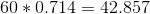. So the trains intersect after 2 hours and about 43 minutes, so at 10:43AM.

To figure out how far from San Francisco they are, figure out how many miles the blue train could have gone in 2.714 hours. In other words, plug 2.714 back into the equation, giving you an answer of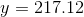.

1 2 3 4 5 7 Next →

### All Algebra 1 Resources